# Three ints

The sum of three consecutive integers is 2016. What numbers are they?

Correct result:

a =  671
b =  672
c =  673

#### Solution:

a+b+c=2016
b = a+1
c = b+1

a+b+c = 2016
a-b = -1
b-c = -1

a = 671
b = 672
c = 673

Our linear equations calculator calculates it.We would be pleased if you find an error in the word problem, spelling mistakes, or inaccuracies and send it to us. Thank you!Tips to related online calculators
Do you have a linear equation or system of equations and looking for its solution? Or do you have quadratic equation?

## Next similar math problems: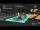The voltage station is every day changing the master password, which consists of three letters. Code generation process does not change and is based on the following procedure: The following letters (A) to (I) correspond to different numbers from 1 to 9.
• Three numbersFind three numbers so that the second number is 4 times greater than the first and the third is lower by 5 than the second number. Their sum is 67.
• Odd numbersThe sum of four consecutive odd numbers is 1048. Find those numbers ...
• Octahedron - sumOn each wall of a regular octahedron is written one of the numbers 1, 2, 3, 4, 5, 6, 7 and 8, wherein on different sides are different numbers. For each wall John make the sum of the numbers written of three adjacent walls. Thus got eight sums, which also
• Self-counting machineThe self-counting machine works exactly like a calculator. The innkeeper wanted to add several three-digit natural numbers on his own. On the first attempt, he got the result in 2224. To check, he added these numbers again and he got 2198. Therefore, he a
• Chickens and rabbitsIn the yard were chickens and rabbits. Together they had 27 heads and 86 legs. How many chickens and how many rabbits were in the yard?
• Four integersFnd four consecutive integers so that the product of the first two is 70 times smaller than the product of the next two.
• n-gon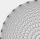Gabo draw n-gon, which angles are consecutive members of an arithmetic sequence. The smallest angle is 70° biggest 170°. How many sides have Gabo's n-gon?
• Freedom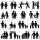In the city are 3/9 of women married for 3/6 men. What proportion of the townspeople is free (not married)? Express as a decimal number.
• Have solutionThe sum of four consecutive even numbers is 92. Determine these numbers.
• Candy and boxesWe have some number of candy and empty boxes. When we put candies in boxes of ten, there will be 2 candies and 8 empty boxes left, when of eight, there will be 6 candies and 3 boxes left. How many candy and empty boxes left when we put candies in boxes of
• Consecutive numbersSum of ten consecutive numbers is 105. Determine these numbers (write first and last).
• Endless lego setThe endless lego set contains only 6, 9, 20 kilograms blocks that can no longer be polished or broken. The workers took them to the gym and immediately started building different buildings. And of course, they wrote down how much the building weighed. The
• Pine's forest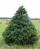There were so many pines in the forest that if they were sequentially numbered 1, 2, 3,. .. , would use three times more digits than the pine trees alone. How many pine trees were there in the forest?
• AM of three numbersThe number 2010 can be written as the sum of 3 consecutive natural numbers. Determine the arithmetic mean of these numbers.
• Logistics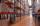Head of logistics department informed the meeting of management of the same item is currently not available in sufficient quantity in all three warehouses of the company. We have the total 135 pieces. It was 37 pieces more in the warehouse No. 3 than in t
• PoolIf water flows into the pool by two inlets, fill the whole for 19 hours. The first inlet filled pool 5 hour longer than the second. How long pool take to fill with two inlets separately?